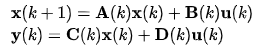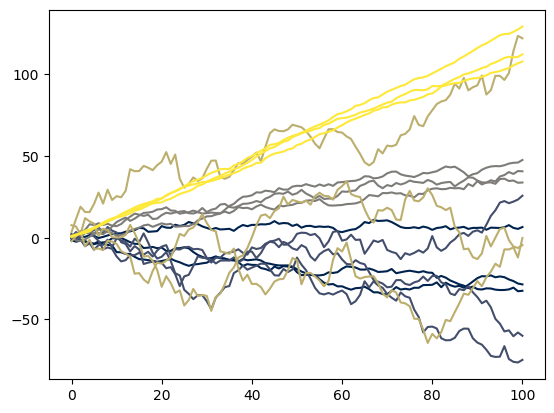# Using pyro.markov for Time-Series Variational Inference

Hi Everyone,

I’m a little new to Pyro, but I’m interested in using its variational inference capabilities to infer the hidden states from time-series models. I’ve been trying to build an example case to understand the tools available for modeling these types of systems, but I’ve gotten stuck in defining the ‘‘model’’ and ‘‘guide’’ functions. I’m working with a standard state-space system of the formMy understanding from the tutorials is that models of this form need to be specified using the ‘‘markov’’ effect handler, but the actual implementation is unclear, particularly when the goal is to infer the hidden states instead of marginalizing them out.

So far I’ve put together this structure in my ‘‘model’’:

``````data_plate = pyro.plate("data", data.shape, dim=-1)
for t in pyro.markov(range(len(data)-1)):
x1[t+1] = pyro.sample("x1_{}".format(t+1), dist.Normal(x1[t] + dt*wn*x2[t], x1_std))
x2[t+1] = pyro.sample("x2_{}".format(t+1), dist.Normal(x2[t] + dt*wn*(-2*zeta*x2[t] - x1[t]), x2_std))

with data_plate:
data[t+1] = pyro.sample("y_{}".format(t+1), dist.Normal( -2*zeta*x2[t+1]-x1[t+1], meas_noise), obs=inp[t+1])
``````

I think that the first section, describing ‘‘x1’’ and ‘‘x2’’, gives the Markovian relationship among the system states and that the second section, describing ‘‘data’’, specifies the conditional independence of the observed quantities given a knowledge of the current state. However, I’m not sure if this understanding is correct, or why these structures (’‘plate’’ and ‘‘markov’’) operate this way in this context. I’m also unsure on how to proceed to form a guide that infers the states ‘‘x1’’ and ‘‘x2’’.

Does anyone have any advice they can share on building these types of models?

1 Like

Hi @ALund. I’ll share a few things and try to clarify some points.

What your model (or the segment of it that you’ve provided does) is a little nonstandard (not that there’s anything wrong with that):

• it posits that there’s a single latent 2d path that evolves for `data.shape - 1` timesteps
• There are `N` noisy observations of this single path (that’s your plate and `y` stuff)

In addition there’s an error with your initialization of `data_plate`; if you try running that, you’ll get

``````TypeError: arange() received an invalid combination of arguments - got (torch.Size, device=torch.device), but expected one of:
* (Number end, *, Tensor out, torch.dtype dtype, torch.layout layout, torch.device device, bool pin_memory, bool requires_grad)
* (Number start, Number end, Number step, *, Tensor out, torch.dtype dtype, torch.layout layout, torch.device device, bool pin_memory, bool requires_grad)
``````

since `plate` wants a single positive integer telling it how many terms in the product there are.

I think that what your model may have been intended to do (and many apologies if you did truly mean the interpretation above!) is the more conventional `N` observations of timeseries of length `T`. These timeseries of length `T` correspond to latent rvs (your `x_1` and `x_2` above) and observed noisy realizations `y`.

Here’s a little model that does exactly that. It’s in 1d and models just a latent random walk and centered state space uncertainty. But it should be pretty clear how you can modify it to meet your needs!

``````def state_space_model(data, N=1, T=2, prior_drift=0., verbose=False):
# global rvs
drift = pyro.sample('drift', dist.Normal(prior_drift, 1))
vol = pyro.sample('vol', dist.LogNormal(0, 1))
uncert = pyro.sample('uncert', dist.LogNormal(-5, 1))

if verbose:
print(
f"Using drift = {drift}, vol = {vol}, uncert = {uncert}"
)

# the latent time series you want to infer
# since you want to output this, we initialize a vector where you'll
# save the inferred values
latent = torch.empty((T, N))

# I think you want to plate out the same state space model for N different obs
with pyro.plate('data_plate', N) as n:
x0 = pyro.sample('x0', dist.Normal(drift, vol))  # or whatever your IC might be
latent[0, n] = x0

# now comes the markov part, as you correctly noted
for t in pyro.markov(range(1, T)):
x_t = pyro.sample(
f"x_{t}",
dist.Normal(latent[t - 1, n] + drift, vol)
)
y_t = pyro.sample(
f"y_{t}",
dist.Normal(x_t, uncert),
obs=data[t - 1, n] if data is not None else None
)
latent[t, n] = x_t

return pyro.deterministic('latent', latent)
``````

A few things to note here.

• We write `data[t - 1, n]`. This is not because we are being acausal but rather because data should be of shape `(N, T - 1)`. So the `t - 1`-th element of `data` should correspond with the `t`-th element of the latent time series. This is because the latent time series must be one element longer as the Markov assumption depends on an initial condition.
• If our goal were actually to infer latent random walks, this is by far not the most efficient way to implement it. `pyro.markov` is fundamentally sequential and thus slow. since a random walk with drift `mu` and volatility `sigma` is a deterministic transformation of white noise, something much quicker would be
``````noise = pyro.sample('noise', dist.Normal(0, 1).expand((T, N)))
random_walk = pyro.deterministic('random_walk', (mu + sigma * noise).cumsum(dim=0))
``````

But this is not what your model does, so I have implemented it using `markov` to make it easier for you to change to meet your needs!

• We wrapped the tensor of all the latent rvs in a `deterministic` so that we can grab it upon model return. This just makes life easier and doesn’t affect the joint density of the model at all.
• Because our `drift`, `vol`, and `uncert` are global rvs, each run of the model generates substantially different-looking sample paths. Check it out:
``````    # draws from the prior predictive are shape (T, N)
# each draw uses different draws from global drift and vol params
n_prior_draws = 5
prior_predictive = torch.stack(
[state_space_model(None, N=N, T=T) for _ in range(n_prior_draws)]
)

colors = plt.get_cmap('cividis', n_prior_draws)
fig, ax = plt.subplots()
list(map(
lambda i: ax.plot(prior_predictive[i], color=colors(i)),
range(prior_predictive.shape)
))
``````Each color corresponds with a different draw from the prior predictive. We drew 5 different draws, each with `N = 3` and `T = 100`.

Now, to your question about fitting these models. Since we have a random variable `x_t` for each `t` in question, it really is not such a bad idea to use one of the built-in `autoguide`s to construct your guide:

``````    guide = pyro.infer.autoguide.AutoDiagonalNormal(state_space_model)
svi = pyro.infer.SVI(state_space_model, guide, optim, loss=pyro.infer.Trace_ELBO())

niter = 2500  # or whatever, you'll have to play with this and other optim params
pyro.clear_param_store()
losses = torch.empty((niter,))

for n in range(niter):
loss = svi.step(data, N=data_N, T=data_T)
losses[n] = loss

if n % 50 == 0:
print(f"On iteration {n}, loss = {loss}")
``````

I will say that I do this all the time and it works quite well.

About sampling from the posterior: no problem. There is a great utility class for this called `pyro.infer.Predictive`:

``````# you can extract the latent time series in a variety of ways
# one of these is the pyro.infer.Predictive class
num_samples = 100
posterior_predictive = pyro.infer.Predictive(
state_space_model,
guide=guide,
num_samples=num_samples
)
posterior_draws = posterior_predictive(None, N=data_N, T=data_T)

# since our model returns the latent, we should have this in the `latent` value
print(
posterior_draws['latent'].squeeze().shape == (num_samples, data_T, data_N)
)
``````

So that is just about all there is that. Hopefully this is at least somewhat helpful. Feel free to reach out if you have any more questions.

3 Likes

Thanks Dave! Your explanation makes the whole process a lot clearer.